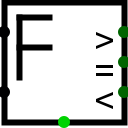#Floating Point Comparator

 Library: Arithmetic Introduced: 3.5 Appearance:## Behavior

This component compares two floating-point values coming in via the west inputs .
and presents three output signals > = <. normally only one of these values is a 1 if an error is detected all outputs are a 0 and and the error output output in the south, which is set to 1 if the component receives an error(E) or undefined(U) signal or the floating-point values NaN

## Pins

West edge, north end:
Input: The first floating-point value to compare. Bit width matches the Data Float size attribute.
West edge, south end:
Input: The second floating-point value to compare. Bit width matches the Data Float size attribute.
East edge: labeled >
Output: 1 if the first input is greater than the second input, 0 if the first input is less than or equal the second input. The bit width is 1.
East edge: labeled =
Output: 1 if the first input equals the second input, 0 if the first input is not equal the second input. The bit width is 1.
East edge: labeled <
Output: 1 if the first input is greater than the second input, 0 if the first input is less than or equal the second input. The bit width is 1.
South edge:
OutPut: Error Set to 1 if the negator receives an error (E) or undefined (U) signal or the float value NaN

## Attributes

When the component is selected or being multiply, Alt-0 throught Alt-9 alter its Float size attribute. Only 32 or 64bits

Float size
The bit width of the values to be multiply and of the result. Only 32 or 64 bits

None.

## Text Tool Behavior

None.

Back to Library Reference